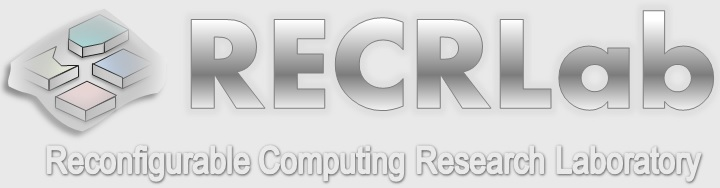Winter 2019 - ECE2700: Digital Logic Design

 Unit 1: Introduction to Logic Circuits Unit 2: Optimized Implementation of Logic Functions Unit 3: Implementation Technology Unit 4: Number Systems and Computer Arithmetic Unit 5: Combinational Circuits Unit 6: Synchronous Sequential Circuits Unit 7: Introduction to Digital System Design Homeworks, Quizzes, Exams Homework 1 Solutions - Homework 1 Quiz 1 Solutions - Quiz 1 Homework 2 Solutions - Homework 2 Quiz 2 Solutions - Quiz 2 MIDTERM Exam Solutions - MIDTERM Exam Homework 3 Solutions - Homework 3 Quiz 3 Solutions - Quiz 3 Homework 4 Solutions - Homework 4 Quiz 4 Solutions - Quiz 4

LABORATORY

Laboratory Assignments

FINAL PROJECT

List of Projects

Reconfigurable Computing Research Laboratory (RECRLab), Electrical and Computer Engineering Department, Oakland University